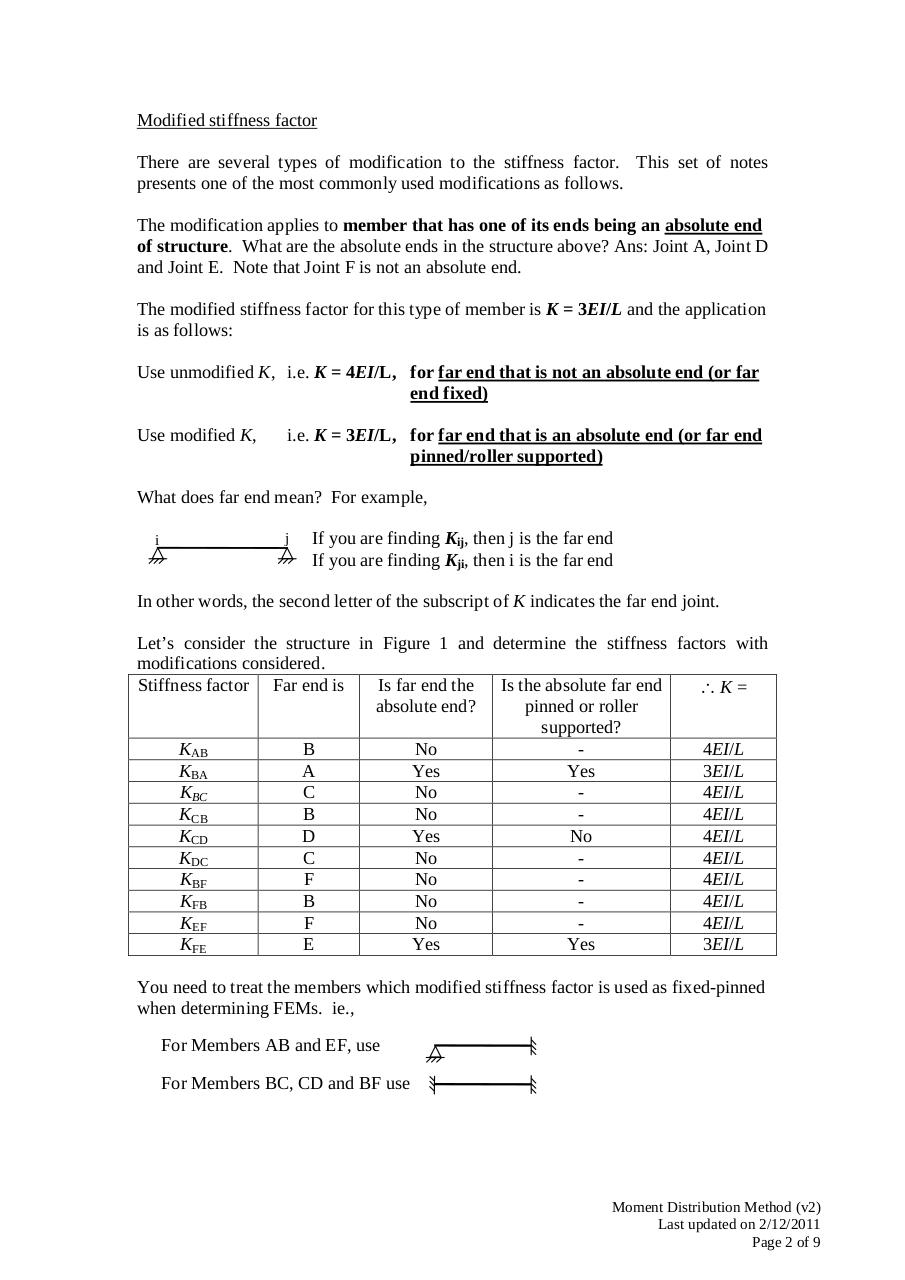# TDII MDM (v2).pdfPage 1 2 3 4 5 6 7 8 9

#### Text preview

Modified stiffness factor
There are several types of modification to the stiffness factor. This set of notes
presents one of the most commonly used modifications as follows.
The modification applies to member that has one of its ends being an absolute end
of structure. What are the absolute ends in the structure above? Ans: Joint A, Joint D
and Joint E. Note that Joint F is not an absolute end.
The modified stiffness factor for this type of member is K = 3EI/L and the application
is as follows:
Use unmodified K, i.e. K = 4EI/L, for far end that is not an absolute end (or far
end fixed)
Use modified K,

i.e. K = 3EI/L, for far end that is an absolute end (or far end
pinned/roller supported)

What does far end mean? For example,
i

j

If you are finding Kij, then j is the far end
If you are finding Kji, then i is the far end

In other words, the second letter of the subscript of K indicates the far end joint.
Let’s consider the structure in Figure 1 and determine the stiffness factors with
modifications considered.
Is far end the Is the absolute far end
Stiffness factor Far end is
∴K=
absolute end?
pinned or roller
supported?
KAB
B
No
4EI/L
KBA
A
Yes
Yes
3EI/L
KBC
C
No
4EI/L
KCB
B
No
4EI/L
KCD
D
Yes
No
4EI/L
KDC
C
No
4EI/L
KBF
F
No
4EI/L
KFB
B
No
4EI/L
KEF
F
No
4EI/L
KFE
E
Yes
Yes
3EI/L
You need to treat the members which modified stiffness factor is used as fixed-pinned
when determining FEMs. ie.,
For Members AB and EF, use
For Members BC, CD and BF use

Moment Distribution Method (v2)
Last updated on 2/12/2011
Page 2 of 9